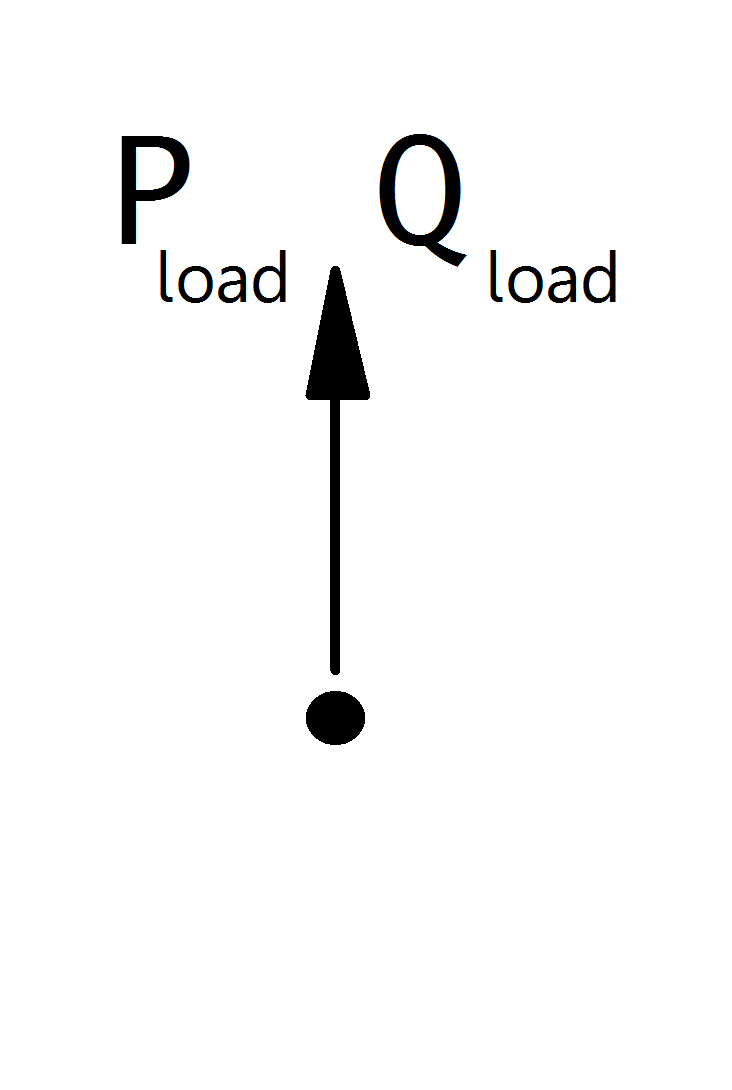# Static Generator¶

## Create Function¶

pandapower.create_sgen(net, bus, p_kw, q_kvar=0, sn_kva=nan, name=None, index=None, scaling=1., type=None, in_service=True, max_p_kw=nan, min_p_kw=nan, max_q_kvar=nan, min_q_kvar=nan, controllable=nan, k=nan, rx=nan)

Adds one static generator in table net[“sgen”].

Static generators are modelled as negative PQ loads. This element is used to model generators with a constant active and reactive power feed-in. If you want to model a voltage controlled generator, use the generator element instead.

All elements in the grid are modelled in the consumer system, including generators! If you want to model the generation of power, you have to assign a negative active power to the generator. Please pay attention to the correct signing of the reactive power as well.

INPUT:

net - The net within this static generator should be created

bus (int) - The bus id to which the static generator is connected

p_kw (float) - The real power of the static generator (negative for generation!)

OPTIONAL:

q_kvar (float, default 0) - The reactive power of the sgen

sn_kva (float, default None) - Nominal power of the sgen

name (string, default None) - The name for this sgen

index (int, None) - Force a specified ID if it is available. If None, the index one higher than the highest already existing index is selected.

scaling (float, 1.) - An OPTIONAL scaling factor to be set customly

type (string, None) - type variable to classify the static generator

in_service (boolean) - True for in_service or False for out of service

max_p_kw (float, NaN) - Maximum active power injection - necessary for controllable sgens in OPF

min_p_kw (float, NaN) - Minimum active power injection - necessary for controllable sgens in OPF

max_q_kvar (float, NaN) - Maximum reactive power injection - necessary for controllable sgens in OPF

min_q_kvar (float, NaN) - Minimum reactive power injection - necessary for controllable sgens in OPF

controllable (bool, NaN) - Whether this generator is controllable by the optimal powerflow

k (float, NaN) - Ratio of nominal current to short circuit current

rx (float, NaN) - R/X ratio for short circuit impedance. Only relevant if type is specified as motor so that sgen is treated as asynchronous motor

OUTPUT:
index (int) - The unique ID of the created sgen
EXAMPLE:
create_sgen(net, 1, p_kw = -120)
pandapower.create_sgen_from_cosphi(net, bus, sn_kva, cos_phi, mode, **kwargs)

Creates an sgen element from rated power and power factor cos(phi).

INPUT:

net - The net within this static generator should be created

bus (int) - The bus id to which the static generator is connected

sn_kva (float) - rated power of the generator

cos_phi (float) - power factor cos_phi

mode (str) - “ind” for inductive or “cap” for capacitive behaviour

OUTPUT:
index (int) - The unique ID of the created sgen

All elements including generators are modeled from a consumer point of view. Active power will therefore always be negative, reactive power will be negative for inductive behaviour and positive for capacitive behaviour.

## Input Parameters¶

net.sgen

 Parameter Datatype Value Range Explanation name string name of the static generator type string naming conventions: “PV” - photovoltaic system “WP” - wind power system “CHP” - combined heating and power system type of generator bus* integer index of connected bus p_kw* float $$\leq$$ 0 active power of the static generator [kW] q_kvar* float reactive power of the static generator [kVar] sn_kva float $$>$$ 0 rated power ot the static generator [kVA] scaling* float $$\geq$$ 0 scaling factor for the active and reactive power max_p_kw** float Maximum active power min_p_kw** float Minimum active power max_q_kvar** float Maximum reactive power min_q_kvar** float Minimum reactive power controllable** bool States if sgen is controllable or not, sgen will not be used as a flexibilty if it is not controllable k*** float $$\geq$$ 0 Ratio of nominal current to short circuit current rx*** float $$\geq$$ 0 R/X ratio for short circuit impedance. Only relevant if type is specified as motor so that sgen is treated as asynchronous motor in_service* boolean True / False specifies if the generator is in service.

*necessary for executing a power flow calculation
**optimal power flow parameter

## Electric Model¶

Static Generators are modelled as PQ-buses in the power flow calculation:The PQ-Values are calculated from the parameter table values as:

\begin{align*} P_{sgen} &= p\_kw \cdot scaling \\ Q_{sgen} &= q\_kvar \cdot scaling \\ \end{align*}

Note

Static generators should always have a negative p_kw value, since all power values are given in the consumer system. If you want to model constant power consumption, please use the load element instead of a static generator with positive active power value. If you want to model a voltage controlled generator, use the generator element.

Note

The apparent power value sn_kva is provided as additional information for usage in controller or other applications based on panadapower. It is not considered in the power flow!

## Result Parameters¶

net.res_sgen

 Parameter Datatype Explanation p_kw float resulting active power demand after scaling [kW] q_kvar float resulting reactive power demand after scaling [kVar]

The power values in the net.res_sgen table are equivalent to $$P_{sgen}$$ and $$Q_{sgen}$$.